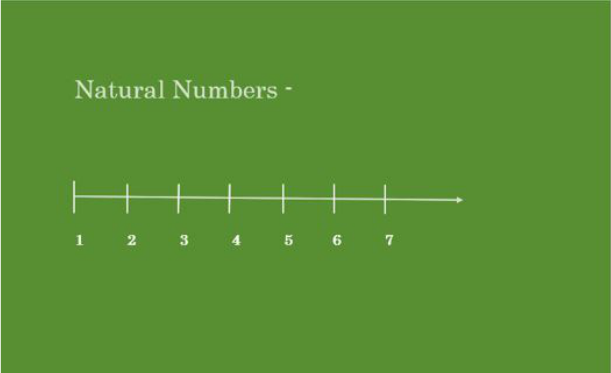# How to identify rational numbers?

• Difficulty Level : Expert
• Last Updated : 05 Oct, 2021

The method to represent and work with numbers is known as the number system. A number system is a system of writing to represent numbers. It is the mathematical notation used to represent numbers of a given set by using digits or other symbols. It has arithmetic operations to perform division, multiplication, addition, and subtraction between numbers. Some important number systems are as follows:

• Decimal Number System
• Binary Number System
• Octal Number System

### Numbers and Digits

Numbers are the counts or measurements used in mathematics, numerals are used to define numbers. A numeral can be defined as a symbol used for counting, for instance, there are 55 books in the library, where 56 is the numeral which is a combination of digits 5 and 6. A digit is a single numeral, the combination of digits form numerals. In the decimal number system, there are 10 digits, they are 0, 1, 2, 3, 4, 5, 6, 7, 8, 9.

### What are Naturals Numbers?

In the Number system, Natural numbers are the numbers that start from 1 and count up to Infinity. For example – (1, 2, 3, 4, 5, 6, 7, 8, 9, 10, 11,12, 13, 14, 15, 16, 17, 18, 19, 20, 21..…….. ∞) are Natural Numbers.### What are Rational Numbers?

A rational number is a number that can be represented in a p/q form such that q is not equal to 0. The set of rational numbers Include positive, negative numbers, and zero and it is denoted by Q. Rational number can also be expressed as a fraction.

When a number is expressed in a p/q form or in fraction form where both the numerator and the denominator part are integers, then the number is known as a rational number.

Some examples of rational numbers are: 1/2, -2/7, 7/10, -7/10, 14/99

The number “0” is also a rational number, as we can represent it in many forms such as 0/1, 0/2, 0/3, etc. But, 1/0, 2/0, 3/0, etc. are not rational numbers because they give us infinite values.

Note: Rational numbers can also be expressed in decimal form.

### Types of Rational Numbers

There are different types of rational numbers and they are:

• Natural Numbers: All natural numbers are rational numbers because they can be written as in p/q form. Like 2 can be expressed in 2/1 (p/q) form.

Example – 1, 2, 3, 4, 5 …. etc.

• Terminating Decimals: Rational numbers can also be expressed in decimal form because decimal numbers can be represented in p/q form. For example, 1.1 can be written as 1.1 = 11/10. Thus all terminating decimals are rational numbers.

Example – ( 0.45, 0.7120, 0.9778 etc. )

• Non-Terminating Decimals: Non-Terminating decimals having repeated numbers after the decimal point such as 0.2222….., 0,12121212…. are also rational numbers. Since 0.222… can be written as 1/2, therefore it is a rational number.

Example – ( 0.2222

230;.., 0.121212…..  etc. )
• Fractions: When a number is expressed in a p/q form or in fraction form where both the numerator and the denominator part are integers, then it is a rational number.

Example – 3/4, 2/7, 7/10, -7/10, 14/99 (all of them are in p/q form)

• Whole Numbers: All whole numbers are rational numbers because the whole numbers can be expressed in p/q fraction form.

Example – 0 is a rational number because it can be written as a fin 0/1, 0/-2,… etc.

### How to identify rational numbers?

There are some conditions to check whether a number is a rational number or not. They are:

1. Always it is represented in the p/q form, where q≠0. For example – 3/4 , 2/7,  7/10, -7/10, 0/1 etc.

2. A rational number can be further simplified and represented in decimal form. For example – 0.9, -0.875, 3.25, -2.0 etc.

### Sample Questions

Question 1. Is 2.2 is a rational number?

Yes 2.2 is a rational number because 2.2 can also be written as p/q form that is 22/10.

Question 2. Identify whether Mixed Fraction 1 5/4 is a Rational Number or Not

trong>

The Simplest Form of Mixed fraction 1 5/4 is 9/4. It is in p/q form. Thus, 9/4 is a Rational Number.

Question 3. How to Identify a Rational Number?

When a number is expressed in the p/q form where p, q are integers and q is non-zero then it called as a Rational Number.

Question 4. Is 12 a Rational Number?

Yes 13 is a Rational Number because it can be expressed in 12/1 that is p/q form.

Question 5. Is 0 a rational number?

Yes, 0 is a rational number because it is an integer and It can be written in p/q form such as 0/1, 0/2, where b is a non-ze

nteger. Hence, 0 is a rational number.

Question 6. Is 1 a Rational Number?

Yes, 1 is a Rational Number because it can be expressed in 1/1 that is p/q form.

Question 7. Which of the following rational numbers are positive?

(i) (-4)/3         (ii) 9/8          (iii) (-14)/(-9)       (iv) (-21)/13